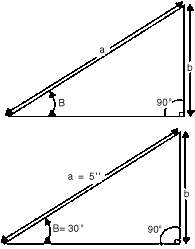NOTE: Setting constants and multipliers are available in Bulletin #818 @ no charge.HOW TO SET UP SINE ANGLE EQUIPMENT1. Setting a sine bar, sine block, sine angle plate or chuck to a given angle is based on solving the shop formula for finding the acute angle "B" of a right triangle: The sine of an angle equals the side opposite divided by the hypotenuse, or2. If you know that angle "B" is 30 and that hypotenuse "a" is 5", the formula is solved by substituting these values in the formula in step 1: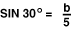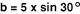Consulting the table of sines found in most any machinist's handbook, the sine of 30 is found to be .500. Substituting this value in the formula,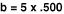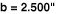3.To set any 5" sine angle tool to 30 , a No. 9118-5 Sine Block for example, follow the same procedure as in step 2. The hypotenuse "a" is 5", the fixed distance between the sine roll centers, and "b" is the required gage block height combination:The answer, b = 2.500", indicates the gage block height required to elevate the sine block to a precise 30o angle.IMPORTANT: When using sine angle equipment with center distance other than 5", be sure to multiply the constant as follows: 20" Center Distance, Multiple Constant by 4 10" Center Distance, Multiply Constant by 2 7" Center Distance, Multiply Constant by 1.4 3" Center Distance, Multiply Constant by 0.6SINE

Automation and Metrology 130 Liberty Street Painesville, Ohio 44077
Phone:  (440) 354 6436  Fax:  (440) 639 9983
E-mail: sales@auto-met.com

www.auto-met.com